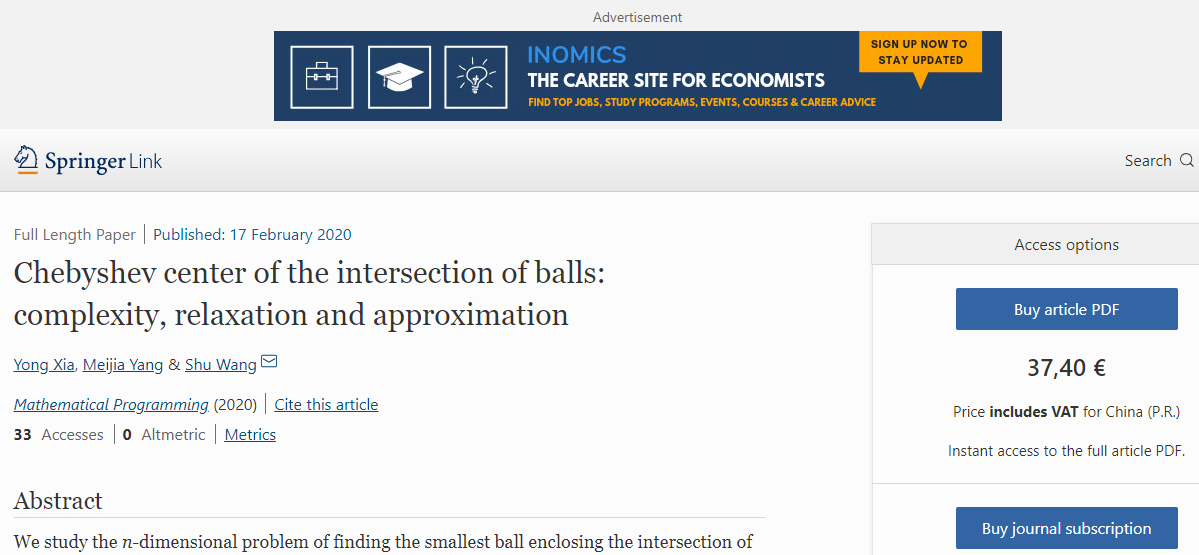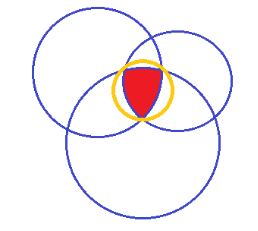﻿ Team of Prof. Xia Yong Publishes Paper in Mathematical Programming-BEIHANG UNIVERSITY中文Home / News & Events / News / Content

# Team of Prof. Xia Yong Publishes Paper in Mathematical Programming

## Release time：March 19, 2020 / Li Mingzhu

A breakthrough was made recently in the field of convex geometry by Prof. Xia Yong, Yang Meijia and Wang Shu, two doctoral students enrolled in 2015 and 2014 respectively. The team from the School of Mathematical Sciences published the research under the title “Chebyshev Center of the Intersection of Balls: Complexity, Relaxation and Approximation” in Mathematical Programming, a noted journal dealing with every aspect of mathematical optimization with an impact factor of 3.785 (2018).The problem which they studied dated back to 1857 when James Joseph Sylvester, a celebrated British mathematician and fellow of the Royal Society, raised the question of finding the circle of smallest radius enclosing a given finite set of points in the plane, or the Chebyshev center of the given points. The general version of the problem is to find the Chebyshev center of a convex set.

In 2007, Amir Beck, a famous expert in optimization and professor of Technion-Israel Institute of Technology whocame upwitha fast iterative shrinkage-thresholding algorithm (FISTA), presented the Chebyshev center problem (CCB) of finding the smallest ball enclosing the intersection of p given balls in the n-dimensional space.The problemhas important applications in fields like robust estimation, wireless network communication, control, optimization, etc. The following figure is an example of the problem when n = 2 and p = 3 (the yellow circle is the smallest one that can cover the red area).Prof. Beck provided a solution to the problem using a standard convex quadratic programming (SQP) method through dual relaxation and proved that the approximation is accurate when pn - 1 in 2007. He changed the sufficient condition into pn in 2009.

The breakthrough made by the team of Prof. Xia concerning this problem is to first prove that (CCB) is NP-hard and discuss the hard case p > n. According to the team, (CCB) is polynomially solvable when either n or pn (>0) is fixed. They also proved that the (SQP) method proposed by Prof. Beck has an approximation ratio of 2 (which means that the function value of the approximate solution is less than two times the accurate minimum) for the first time.

This research was supported by National Science Fund for Excellent Young Scholars, National Natural Science Foundation of China, and Beijing Natural Science Foundation.